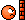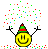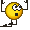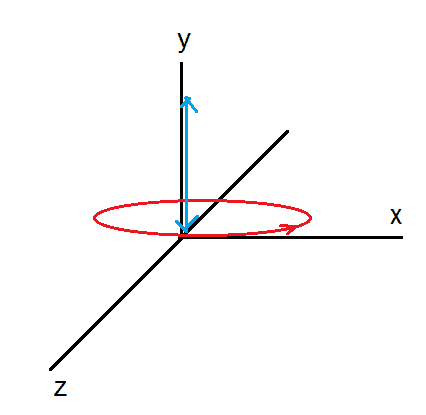# Charged particle in Electric and Magnetic field

• Vibhor

## Homework Statement

A particle of specific charge q/m is projected from the origin of coordinates with initial velocity ##v_1\hat{i}+v_2\hat{j}## in space having uniform electric field and magnetic field as ##-E\hat{j}## and ##-B\hat{j}## respectively. The particle will definitely return to the origin once if

A) ##\frac{v_1B}{\pi E}## is an integer
B) ##\frac{v_2B}{\pi E}## is an integer
C) ##\frac{\sqrt{{v_1}^2+{v_2}^2}B}{\pi E}## is an integer
D) ##\frac{q}{m}\frac{\sqrt{{v_1}^2+{v_2}^2}B}{\pi E}## is an integer

## Homework Equations

Lorentz force = ##q\vec{v}## x ##\vec{B} + q\vec{E}##

## The Attempt at a Solution

The force on the charged particle will be ##\vec{F} = qBv_y\hat{i} - qE\hat{j}- qBv_x\hat{k}## .

There will be a force on the particle in negative z- direction which means particle will never return to origin . I am not sure how to move forward .

Thanks

Last edited:

There will be a force on the particle in negative z- direction
If so, it acquires a velocity component in that direction and your Lorentz force changes !

If so, it acquires a velocity component in that direction and your Lorentz force changes !

Ok . So what should be my next step ?

What kind of trajectories can you think of in a field like that ? Which would let the partcle go through the origin more than once ?

Sorry , I have no idea .

## Homework Statement

A particle of specific charge q/m is projected from the origin of coordinates with initial velocity ##v_1\hat{i}+v_2\hat{j}## in space having uniform electric field and magnetic field as ##-E\hat{j}## and ##-B\hat{j}## respectively. The particle will definitely return to the origin once if

## Homework Equations

Lorentz force = ##q\vec{v}## x ##\vec{B} + q\vec{E}##

## The Attempt at a Solution

The force on the charged particle will be ##\vec{F} = qBv_y\hat{i} - qE\hat{j}- qBv_x\hat{k}## .
Check the vector product. Y component of velocity does not give component of force with y component of magnetic field.
There will be a force on the particle in negative z- direction which means particle will never return to origin .
Thanks
Without the electric field, the particle would move in the ( x,z) plane, perpendicular to the magnetic field, along a circle.
Recall that ##\vec F= m \frac {d \vec v }{dt}##. Write it in components.

Last edited:
•Vibhor
Hello ehild ,

Check the vector product. Y component of velocity does not give component of force with y component of magnetic field.

Sorry . That was a typo . ##\vec{F} = qBv_z\hat{i} - qE\hat{j}- qBv_x\hat{k}##

Without the electric field, the particle would move in the ( x,z) plane, perpendicular to the magnetic field, along a circle.

Why ? There is an initial component of velocity along Y-axis .

Recall that ##\vec F= m \vec {\dot v}##. Write it in components.

##m a_x = qBv_z##
##m a_y = -qE##
##m a_z = -qBv_x##

Hello ehild ,

Why ? There is an initial component of velocity along Y-axis .
Ah, yes.

##m a_x = qBv_z##
##m a_y = -qE##
##m a_z = -qBv_x##
Write the acceleration components as time derivatives of the velocity components, and solve the system of differential equation.

•Vibhor
Write the acceleration components as time derivatives of the velocity components, and solve the system of differential equation.
You mean I should solve the following three equations .

$$m \frac{dv_x}{dt} = qBv_z$$
$$m \frac{dv_y}{dt} = -qE$$
$$m \frac{dv_z}{dt} = -qBv_x$$

They look bit difficult to solve ?

You mean I should solve the following three equations .

$$m \frac{dv_x}{dt} = qBv_z$$
$$m \frac{dv_y}{dt} = -qE$$
$$m \frac{dv_z}{dt} = -qBv_x$$

They look bit difficult to solve ?

No. You can solve the y component at once. The x, z components correspond to circular motion. (Why?) Take the derivative of the first equation and substitute ## \frac{dv_z}{dt} ## into the third one.

•Vibhor
Ok . So what should I do once I get vx,vy,vz ? How do I impose the condition that particle passes the origin ? Should I need to find general coordinates of the particle ?

Ok . So what should I do once I get vx,vy,vz ? How do I impose the condition that particle passes the origin ? Should I need to find general coordinates of the particle ?
Integrate the velocity components to get the coordinates with the initial condition x=y=z = 0. Find the condition that they are the same at a later time.
No need to use other coordinates.

•BvU and Vibhor
Find the condition that they are the same at a later time.

What is same at a later time ?

What is same at a later time ?
x and y and z all are zero again.

•Vibhor
Ok . I tried finding vx . ##v_x = ±v_1sin(ωt+φ)## where ω = qB/m and φ = nπ/2 ( n is odd) . Is this alright ?

Ok . I tried finding vx . ##v_x = ±v_1sin(ωt+φ)## where ω = qB/m and φ = nπ/2 ( n is odd) . Is this alright ?
You can write it as vx=v1 cos (wt). And what is vz?

•Vibhor
You can write it as vx=v1 cos (wt).

Last edited:
You left your solution with an arbitrary odd n. Choose n =1, then vx=v1cos(wt) will fulfill the initial condition.

•Vibhor
Ok .

So, ##x=\frac{v_1}{ω}sinωt## ??

Ok .

So, ##x=\frac{v_1}{ω}sinωt## ??

•Vibhor
The general coordinates are ##x=\frac{v_1m}{qB}sinωt## , ##y = v_2t - \frac{qE}{2m}t^2## , ##z = \frac{v_1m}{qB}(1-cosωt)##

Last edited:
The general coordinates are ##x=\frac{v_1m}{qB}sinωt## , ##y = v_2t - \frac{qE}{2m}t^2## , ##z = \frac{v_1m}{qB}(1-cosωt)##
Are you sure in the minus sign in z? Check the acceleration.

Then find the time when x, y, z are zero and the condition that this can happen.

•Vibhor

You mean there should be a minus sign ??

You mean there should be a minus sign ??
No, in z. I think the minus sign is at the wrong place. Check.

•Vibhor
Sorry . I think It should be ##x=\frac{v_1m}{qB}sinωt## only . I don't see why there should be a minus sign .

Sorry . I think It should be ##x=\frac{v_1m}{qB}sinωt## only . I don't see why there should be a minus sign .
x is correct. I meant z, sorry.

•Vibhor
##z = - \frac{v_1m}{qB}(1-cosωt) ## ??

##z = - \frac{v_1m}{qB}(1-cosωt) ## ??
Yes.

•Vibhor
Ok .

x will be 0 when ##\frac{qBt}{\pi m }## is an integer , y will be 0 at ##t = \frac{2mv_2}{qE}## , z will be 0 when ##\frac{qBt}{2\pi m }## is an integer

Ok .

x will be 0 when ##\frac{qBt}{\pi m }## is an integer , y will be 0 at ##t = \frac{2mv_2}{qE}## , z will be 0 when ##\frac{qBt}{2\pi m }## is an integer
What integer is it? When is z = 0? And all of them should be zero at the same time.

•Vibhor
Option B)Option B)Correct. Was it difficult?

•Vibhor
Was it difficult?

It surely was until you took over. Honestly speaking , I had almost made up my mind to leave this problem as I was completely clueless. But last couple of hours of problem solving were pretty exciting,thanks to you .

You are amazing.

It was the combination of a circular motion in the x,z plane and a vertical projectile motion in the direction of y. The period of the circular motion should be the same as the flight time of the vertical projectile.Thank you for the thanksLast edited:
•Vibhor